# Z9–I–4 MO 2017

Numbers 1, 2, 3, 4, 5, 6, 7, 8 and 9 were prepared for a train journey with three wagons. They wanted to sit out so that three numbers were seated in each carriage and the largest of each of the three was equal to the sum of the remaining two. The conductor said that it is not a problem, and tried to help the numbers. On the contrary, the dispatcher claimed that it was not possible. Decide who is right.

Result

x = (Correct answer is: V)#### Solution:We would be pleased if you find an error in the word problem, spelling mistakes, or inaccuracies and send it to us. Thank you!

Showing 1 comment:Dr Math
Dispatcher is right. In each wagon shall be the sum of the numbers even. In one wagon must sit 9 and therefore the next two numbers whose sum is 9 and overall sum in the wagon is 18. The total sum of all numbers is 45 and the other two wagons already account for 45-18 = 27. However even number 27  can not be decomposed into the sum of two even numbers (in each wagon, the sum is always even). And so the task is not a solution and dispatcher's right.

2 years ago  1 Like## Next similar math problems:

• Pet store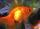In a pet store, they are selling out the fish from one aquarium. Ondra wanted half of all fish, but they don't wish cut by hal fany fish he got one more than demanded. Matthew wished the remaining half of the fish, but as Andrew got half the fish more tha
• Quiz or testI have a quiz with 20 questions. Each question has 4 multiple choice answers, A, B, C, D. THERE IS NO WAY TO KNOW THE CORRECT ANSWER OF ANY GIVEN QUESTION, but the answers are static, in that if the "correct" answer to #1 = C, then it will always be equal
• Amazing numberAn amazing number is name for such even number, the decomposition product of prime numbers has exactly three not necessarily different factors and the sum of all its divisors is equal to twice that number. Find all amazing numbers.
• Unknown numberUnknown number is divisible by exactly three different primes. When we compare these primes in ascending order, the following applies: • Difference first and second prime number is half the difference between the third and second prime numbers. • The prod
• Six-digit primesFind all six-digit prime numbers that contain each one of digits 1,2,4,5,7 and 8 just once. How many are they?
• Four-digit numbers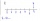Find four-digit numbers where all the digits are different. For numbers, the sum of the third and fourth digits is twice the sum of the first two digits, and the sum of the first and fourth digits is equal to the sum of the second and third digits. The di
• Tunnels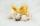Mice had built an underground house consisting of chambers and tunnels: • each tunnel leading from the chamber to the chamber (none is blind) • from each chamber lead just three tunnels into three distinct chambers, • from each chamber mice can get to any
• Toy carsPavel has a collection of toy cars. He wanted to regroup them. But in the division of three, four, six, and eight, he was always one left. Only when he formed groups of seven, he divided everyone. How many toy cars have in the collection?
• MO C–I–1 2018An unknown number is divisible by just four numbers from the set {6, 15, 20, 21, 70}. Determine which ones.
• RemainderA is an arbitrary integer that gives remainder 1 in the division with 6. B is an arbitrary integer that gives remainder 2 the division by. What makes remainder in division by 3 product of numbers A x B ?
• DivisorsThe sum of all divisors unknown odd number is 2112. Determine sum of all divisors of number which is twice of unknown numbers.
• Primes 2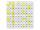Which prime numbers is number 2025 divisible?
• NumberWhat number should be placed instead of the asterisk in number 702*8 to get a number divisible by 6?
• Sum of two primesChristian Goldbach, a mathematician, found out that every even number greater than 2 can be expressed as a sum of two prime numbers. Write or express 2018 as a sum of two prime numbers.
• Divisibility 2How many divisors has integer number 13?
• Multiples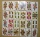What is the sum of the multiples of number 7 that are greater than 30 but less than 56?
• SummandOne of the summands is 145. The second is 10 more. Determine the sum of the summands.